# A New Model to Predict the Global Solar Radiation GSR of Souk-Ahras City

A New Model to Predict the Global Solar Radiation GSR of Souk-Ahras City
Department of Material Sciences, Matter and Radiation Physics Laboratory Mohamed Chérif Messaidia University of Souk Ahras, Road of Annaba, Souk Ahras 41000, Algeria

Department of Electrical Engineering, Mines Laboratory LM, University Larbi Tebessi, Tebessa 12002, Algeria

Corresponding Author Email:
dibdjalel@gmail.com
Page:
67-76
|
DOI:
https://doi.org/10.18280/ejee.240201
12 March 2022
|
Accepted:
21 April 2022
|
Published:
30 April 2022
| Citation

OPEN ACCESS

Abstract:

The value of the global solar radiation GSR reaching the earth is very important because it is an essential variable for different applications. Unfortunately, solar radiation measurements are not available, most of the time, in developing countries because of the lack of measurement means. Moreover, these measurements are difficult to obtain under complicated weather conditions. Thus, solar radiation evaluation models are used. In this study, a new semi-empirical model for the estimation and prediction of the global solar radiation of Souk Ahras area in Algeria is proposed. The model developed is based on meteorological data such as daily hourly temperatures and average relative humidity required from several stations and databases, over a period of four years. The values of the regression coefficients a, b and c calculated are 0.0142, -10.6206 and 57.8367 respectively. To set the modal valid, we have applied it to 10 Algerian cities and we calculated the H/H0 ratio for each site from our model. They have been then compared with the values from the (CDER). We can conclude that our new model gives a good estimate of the average daily global solar radiation (H) for the studied regions (error between 2.49% and 8.93%) and can also be used elsewhere in areas with the same climatic conditions.

Keywords:

solar energy, global solar irradiance, modeling, prediction

1. Introduction

In Algeria, energy needs are totally dependent on fossil fuels. The entire economy of the country is based on the export of these products. Unfortunately, with the fall in oil prices in recent years and the tear sounded by scientists and world leaders (Paris agreement) for the protection of the environment and climate from the adverse effects of excessive use of energy conventional. Our country is forced to move towards other energy alternatives such as the use of renewable energies.

As a reaction and a contribution to the world policy for the preservation of the environment, the Algerian government has proposed several strategies for the reduction of the greenhouse effect. Hereby, we can clearly mention the Horizon 2030 project  that is all based on the partial substitution (total in the long term) of fossil energy by renewable energies, in particular solar energy given the enormous deposit that Algeria possesses. Several attractive projects have been set up and investors have been encouraged to invest in the technological promotion of renewable energies, for example the project SOLAR .

Therefore, information about local solar radiation is considered necessary and important for many applications, including architectural designs and solar energy systems. However, in many developing countries, data on solar energy, unfortunately, are not always available due to the lack of station measuring the parameters of incident solar radiation because of the cost of measuring equipment and their maintenance. Therefore, methods must be devised to estimate solar radiation. Based on meteorological data, many experimental models have been developed to calculate global solar radiation GSR using different climates (Figure 1). Parameters include extra-terrestrial radiation, sunshine hours, average temperature, relative humidity, altitude, longitude, latitude, clouds and radiation, which are measured.

## 1.pngFigure 1. Global solar radiation by EUMETSAT 2019

Solar energy is one of the most important forms of renewable energy, which has become the center of interest of several countries in the world because of its enormous potential especially in the countries of the southern hemisphere as for the 'Algeria. In addition to being clean energy that does not pollute the environment, it is free and accessible to everyone. The aim of this research is to develop a modern method for calculating the amount of total radiation received every hour from sunrise to sunset based on a numerical calculation using mathematical relationships on a horizontal surface located in the Souk Ahras area, which is a city located in the extreme north east of the country, on the Tunisian border, its area is 812 km2. Its location is as follows:

Geographical coordinates :

- Latitude 36° 17′ 15″ north,

- Longitude 7° 57′ 15″ east,

- Altitude 653 m.

The climate of Souk Ahras is a Mediterranean hot-summer climate according to the Köppen-Geiger (Csa) classification .

## 2.pngFigure 2. Solar of radiation components

The method used in our work, to calculate the global solar radiation, depends on a set of factors related to the geographical area of the place studied such as the angle of longitude, the width, the altitude, the relative humidity and the temperature.

The intensity of the external solar radiation decreases as it passes through the Earth’s atmosphere . The reason for the decrease in the intensity of the external solar radiation in the sixth month is the fact that the Earth is located at the aphelion point relative to the Sun, which is the farthest point of the Earth from the Sun, or its height in the first and twelfth months, due to the fact that the Earth is located in Perigee, which is the closest point to the sun and reaches the Earth, three types of solar radiation represent 50% of solar radiation.

It is the rays that reach directly from the sun to the earth, and it represents a large percentage on sunny days, about 27% . On cloudy days, the sun is almost non-existent radiation, when the scattered solar radiation is the majority in this case .

This radiation comes from different parts of the sky due to the presence of clouds, water vapor, the ozone layer. The amount of this radiation is 10% in this case when the sky is clear and 100% when the sky is cloudy [7, 8].

The amount of radiation reflected from the ground and reaching the surface of the solar panels depends on the ground reflection, which is known as albedo. The Albedo (reflectivity) is the energy reflection factor of a surface subjected to solar radiation. This coefficient is dimensionless and represents the ability of a surface to reflect solar radiation. A= Reflected solar flux / incident solar flux, and its value ranges between 0.2 for the usual case and 0.7 when there is snow, and this amount constitutes 13% of the total incoming solar radiation [7, 8]. The Figure 2 shows the types of solar radiation connecting to a tilted solar panel roof.

In general, the amount and intensity of solar radiation are affected by all the previous types by several factors, including: the state of the sky in terms of its clarity and in the case of clouds, the four seasons, the height above sea level, the position of the sun in the sky, the angle of inclination of the sun and the thickness of the atmosphere in addition to the solar angles.

3. Review of Existing Global Radiation Models

The Site and Data source Souk-Ahras city

Description of the site and data source Souk Ahras is a semi-continental and humid region located in eastern Algeria, about 640 km east of the capital (Figure 3). The measured data on solar radiation used in this study were collected over a period of 4 years (2017-2020) by a radiometric station of the Renewable Energy Development Center presented in (Figure 4) with high resolution, installed on the roof of the solar radiation laboratory, based on the weather conditions of the day which are described by several parameters, namely: pressure, temperature, humidity, wind direction and speed, precipitation, cloudy conditions and fog, etc., at the surface and at altitude of the given location in addition to measurements of global solar radiation (GSR) on horizontal surface, solar direct normal irradiance (DNI) and diffuse solar radiation (DSR) on the horizontal plane.

 N° Name of Model Analytic Model N° Name of Model Analytic Model 01 Glover and McCulloch (1958)  $\frac{H}{H_{0}}=0.29 \cos \emptyset+0.52 \frac{S}{S_{0}}$ 02 Page (1961)  $\frac{H}{H_{0}}=0.23+0.48\left(\frac{S}{S_{0}}\right)$ 03 Swartman and Ogunlade (1967)  $\frac{H}{H_{0}}=a+b\left(\frac{S}{S_{0}}\right)+c(R H)$ 04 Iqbal  (1979)  $\frac{H_{d}}{H}=0.791-0.635\left(\frac{S}{S_{0}}\right)$ $\frac{H_{d}}{H}=0.163-0.478\left(\frac{S}{S_{0}}\right)-0.655\left(\frac{S}{S_{0}}\right)^{2}$ 05 Kholagi et al. (1983)  $\frac{H}{H_{0}}=0.191+0.571\left(\frac{S}{S_{0}}\right)$ $\frac{H}{H_{0}}=0.297+0.432\left(\frac{S}{S_{0}}\right)$ $\frac{H}{H_{0}}=0.262+0.454\left(\frac{S}{S_{0}}\right)$ 06 Benson et al. (1984)  $\frac{H}{H_{0}}=0.18+0.60\left(\frac{S}{S_{0}}\right)$ for Jan, Mar and Oct $\frac{H}{H_{0}}=0.24+0.53\left(\frac{S}{S_{0}}\right)$ for Apr and Sep 07​ Ogelman et al. (1984)  $\frac{H}{H_{0}}=0.195-0.676\left(\frac{S}{S_{0}}\right)-0.142\left(\frac{S}{S_{0}}\right)^{2}$ 08 Bahel et al. (1986)  $\frac{H}{H_{0}}=0.175+0.552\left(\frac{S}{S_{0}}\right)$ 09 Bahel et al. (1987)  $\frac{H}{H_{0}}=0.16-0.87\left(\frac{S}{S_{0}}\right)-0.61\left(\frac{S}{S_{0}}\right)^{2}$ $+0.34\left(\frac{S}{S_{0}}\right)^{3}$ 10 Gopinathan (1988)  $\frac{H}{H_{0}}=(-0.309+0.539 \cos \emptyset-0.0693 Z+$$\left.0.290\left(\frac{S}{S_{0}}\right)\right)+(1.527-1.027 \cos \emptyset+$$\left.0.0926 Z-0.359\left(\frac{S}{S_{0}}\right)\right)\left(\frac{S}{S_{0}}\right)$ 11 Newland (1989)  $\frac{H}{H_{0}}=0.34+0.40\left(\frac{S}{S_{0}}\right)+0.17 \log \left(\frac{S}{S_{0}}\right)$ 12 Alsaad (1990)  $\frac{H}{H_{0}}=0.174+0.615\left(\frac{S}{S_{0}}\right)$ 13 Akinoğlu and Ecevit (1990)  $\frac{H}{H_{0}}=0.145-0.845\left(\frac{S}{S_{0}}\right)-0.280\left(\frac{S}{S_{0}}\right)^{2}$ 14 Samuel (1991)  $\frac{H}{H_{0}}=-0.14+2.52\left(\frac{S}{S_{0}}\right)-3.71\left(\frac{S}{S_{0}}\right)^{2}$$+2.24\left(\frac{S}{S_{0}}\right)^{3} 15 Lewis (1992)  \frac{H}{H_{0}}=0.14+0.57\left(\frac{S}{S_{0}}\right) \frac{H}{H_{0}}=0.81-3.34\left(\frac{S}{S_{0}}\right)+7.38\left(\frac{S}{S_{0}}\right)^{2}$$-4.51\left(\frac{S}{S_{0}}\right)^{3}$ 16 Aksoy (1997)  $\frac{H}{H_{0}}=0.148+0.668\left(\frac{S}{S_{0}}\right)-0.079\left(\frac{S}{S_{0}}\right)^{2}$ 17 Ampratwum and Dorvlo (1999)  $\frac{H}{H_{0}}=0.6376+0.2490 \log \left(\frac{S}{S_{0}}\right)$ 18 Chegaar and Chibani (2001)  $\frac{H}{H_{0}}=0.309+0.368\left(\frac{S}{S_{0}}\right)$ For Algiers and Oran $\frac{H}{H_{0}}=0.367+0.367\left(\frac{S}{S_{0}}\right)$ For Beni Abbas $\frac{H}{H_{0}}=0.233+0.591\left(\frac{S}{S_{0}}\right)$ For Tamanrasset 19 Akpabio and Etuk (2003)  $\frac{H}{H_{0}}=0.23+0.38\left(\frac{S}{S_{0}}\right)^{2}$ 20 Ahmad and Ulfat (2004)  $\frac{H}{H_{0}}=0.324+0.405\left(\frac{S}{S_{0}}\right)$ $\frac{H}{H_{0}}=0.348+0.320\left(\frac{S}{S_{0}}\right)-0.070\left(\frac{S}{S_{0}}\right)^{2}$ 21 Chen et al. (2004)  $\frac{H}{H_{0}}=a\left(T_{\max }-T_{\min }\right)^{0.5}+b$ 22 Bakirci (2007)  \begin{aligned} \frac{H}{H_{0}}=0.6307 &-0.7251\left(\frac{S}{S_{0}}\right) \\ &+1.2089\left(\frac{S}{S_{0}}\right)^{2} \\ &-0.4633\left(\frac{S}{S_{0}}\right)^{3} \end{aligned} 23 Bakirci (2009)  $\frac{H_{c}}{H_{0}}=0.6716+0.0760\left(\frac{S}{S_{0}}\right)$ $c \frac{H_{c}}{H_{0}}=0.5622+0.5444\left(\frac{S}{S_{0}}\right)$$-0.4490\left(\frac{S}{S_{0}}\right)^{2}$ 24 Olayinka (2011)  $\frac{H}{H_{0}}=a_{1}+a_{2} T_{\max }+a_{3} \sigma$ 25 Adaramola (2012)  $\frac{H}{H_{0}}=a_{1}+a_{2} T_{m}$ $\frac{H}{H_{0}}=a_{1}+a_{2} \frac{T_{\min }}{T_{\max }}$ 26 Adhikari et al. (2013)  $\frac{H}{H_{0}}=a+b \frac{S}{S_{0}}+c \frac{T_{a v g}}{T_{\max }}+d \ln (R H)$ 27 Li et al. (2013)  $\frac{H}{H_{0}}=a_{1}\left(1+a_{2} R H\right) \Delta T$ 28 Hassan et al. (2016)  $\frac{H}{H_{0}}=a_{1}+a_{2} \Delta T^{0.5}$ 29 Jahani et al. (2017)  $\frac{H}{H_{0}}=a_{1}+a_{2} \Delta T+a_{3} \Delta T^{2}+a_{4} \Delta T^{3}$ 30 Fan et al. (2018)  $\frac{H}{H_{0}}=a_{1}+a_{2} \Delta T+a_{3} \Delta T^{0.25}+a_{4} \Delta T^{0.5}$ \begin{aligned} \frac{H}{H_{0}}=a_{1}+a_{2} \Delta T &+a_{3} \Delta T^{0.25}+a_{4} \Delta T^{0.5} \\ &+a_{5} \frac{T_{m}}{H_{0}} \end{aligned} 31 Ekici and Teke (2018)  $\frac{H}{H_{0}}=a_{1}\left[a_{2}\left(1-e^{(\tau / R H}\right)^{a_{3}}\right)+a_{4}(\tau / R H)^{a_{5}}$ $+a_{6} \cos \left(a_{7} \tau / R H\right)$ $+a_{8} \cos \left(a_{7} T_{\min } / \Delta T\right)$ $\left.+a_{9} \sin \left(a_{7} T_{\min } / \Delta T\right)\right]$ 32 Yildirim et al. (2018)  $\frac{H}{H_{0}}=a_{1}+a_{2} R H+a_{3} \sigma+a_{4} \sigma^{2}+a_{5} \sigma^{3}$

The station consists of two parts:

• The stator consists of EKO MS-64 thermometers for measuring global solar radiation on a horizontal surface (shortwave sensitivity is 7.0 (mV / kW / m2)) and on an inclined surface at the latitude of the site.

• A moving part capable of tracking the path of the sun from sunrise to sunset.

The last one consists of an EKO MS-101D thermometer with a short wave sensitivity of 6.71 (mV/kW/m2), which is pointed at the sun disk to measure the DNI component. Another thermometer EKO MS-64 with a short-wave sensitivity of 7.0 (mV / kW / m2) used for measuring diffuse solar radiation on the horizontal plane. It is equipped with a shade bar to mask the radiation flux coming directly from the sun.

## 3.pngFigure 3. Souk Ahras site location

## 4.png## 5.png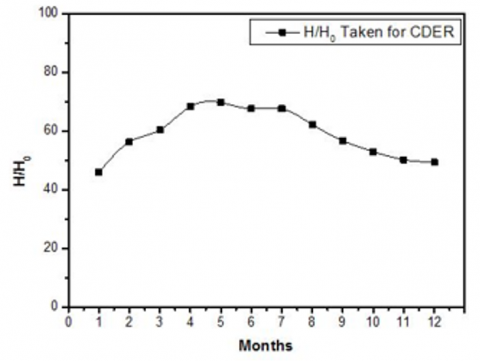Figure 5. H/H0 taken for CDER

All solar components are made with a five-minute interval for each component. (Figure 5) shows the variation of H / H0 changes in terms of months measured in the Souk Ahras region. With H is the monthly average daily global irradiation on a horizontal surface (W/m2 day) and H0 is the monthly average the daily extraterrestrial irradiation (W/m2).

4. Proposed Model

In the literature, several types of models exist for the evaluation and prediction of global solar radiation, the difference between them is the parameters that come into play. These parameters depend on the characteristics and climatic specificities of the region considered. In our case we used the regression equation which depends of temperature and relative humidity. They are the two main parameters that influence the climate of Souk Ahras, this semi-empirical relationship is based on statistical methods applied to available data. Our objective is to find a, b and c that represent the determinants of the matrix for the first, second and third column, respectively, in the following form:

$\frac{H}{H_{0}}=a T^{2}+b R H+c$          (1)

$H_{0}$ can be computed from the following equation $$ :

$H_{0}=\frac{24}{\pi} I_{s c}\left[\cos \emptyset \cos \delta \sin w_{s}+\frac{\pi}{180} w_{s} \sin \emptyset \sin \delta\right]$           (2)

${\left[1+0.033 \cos \frac{360 n}{365}\right] }$

where:

$I_{s c}$ represents the solar constant $\left(I_{s c}=1367 \mathrm{~W} / \mathrm{m}^{2}\right), \emptyset$ is the latitude of the site, $\delta$ presents the solar declination, $w_{s}$ is themean sunrise hour angle for the given month and $n$ is the number of days of the year starting from first January. The solar declination $\delta$ and the mean sunrise hour angle $w_{s}$ can be calculated by Eqns. (3) and (4), respectively :

$\delta=23.45 \sin \left(\frac{360(284+n)}{365}\quad\right)$           (3)

$w_{s}=\cos ^{-1}(\tan \emptyset \tan \delta)$      (4)

$S_{0}=\frac{2}{15} w_{s}$          (5)

We conducted a study for the year 2020, taking into consideration the average values of temperatures T and the percentages of the average values of relative humidity RH for each season (three months) of the year. These values are taken from Renewable Energy Development center (CDER)  and Global Surface Summary of Day (GSOD) .

We calculated the mean values for each day of the month of the Souk Ahras area, for example the month of October, by taking the mean of each day $T_{j}$ with: $T_{j}=\frac{T_{\max }-T_{\min }}{2}$, for more precision. Then, we take the average of the temperaturevalues for the whole month $T_{m}$ with $T_{m}=\sum_{j=1}^{=31} \frac{T_{j}}{31}$. We reproduce the same work for the months of November and December. After that, we calculate the midpoint of temperature $T$ with $T=$ $\sum_{m=1}^{m=3} \frac{T_{m}}{3}$ for the three months we have chosen. Hourly temperature values and relative humidity values for each day of the month are taken from GSOD. As for the value of the average solar radiation of the area H is taken from the data of the CDER. For the solar radiation on the ground H0is calculated from Eq. (2) for the three months considered.

The same work is done for the other chosen quarters, namely January, February, March and July, August and September (we chose three quarters in the year because we have three unknown determinants in our proposed model). Our results are shown in Table 1.

Table 1. Results for T, T², RH, H, H0 and H/H0

 T [°C] T2 [°C] RH H)W/m2) H0)W/m2) H/H0 9.8 96.04 0.697 8588 165.8 51.80 27.5 756.25 0.583 11081 177.60 62.4 9.1 82.81 0.845 8012 160.10 50.04

To solve our system of equations of order 3, we used Cramer's rules for a system of order 3. This method, which is valid when the system has a unique solution, allows to define our determinants as follows:

$\left\{\begin{array}{l}\frac{2401}{25} a+\frac{697}{1000} b+c=\frac{259}{5} \\ \frac{3025}{4} a+\frac{583}{1000} b+c=\frac{312}{5} \\ \frac{8281}{100} a+\frac{169}{200} b+c=\frac{1251}{25}\end{array}\right.$      (6)

$a=0.0142$       (7a)

$b=-10.6206$          (7b)

$c=57.8367$         (7c)

Using the results our model will have the following form:

$\frac{\boldsymbol{H}}{\boldsymbol{H}_{0}}=0.0142 \boldsymbol{T}^{2}-10.6206 \boldsymbol{R} \boldsymbol{H}+57.8367$               (8)

Table 2. Calculated H of the area of Souk Ahras for 2017

 Month T[°C] T²[°C]2 RH C H/H0 1.Jan 5.62 31.5844 0.705 57.8367 50.79768 2.Feb 10.3 106.09 0.735 57.8367 51.53704 3.Mar 12.46 155.2516 0.661 57.8367 53.02106 4.Apr 14.54 211.4116 0.662 57.8367 53.80791 5.May 19.09 364.4281 0.491 57.8367 57.79686 6.jun 26.2 686.44 0.474 57.8367 62.54998 7.Jul 29.55 873.2025 0.341 57.8367 66.61455 8.Aug 29.06 844.4836 0.369 57.8367 65.90937 9.Sep 22.55 508.5025 0.492 57.8367 59.8321 10.Oct 17.24 297.2176 0.61 57.8367 55.57862 11.Nov 11.64 135.4896 0.636 57.8367 53.00595 12.Dec 7.84 61.4656 0.754 57.8367 50.70158 Year 2017

Table 3. Calculated H of the area of Souk Ahras for 2018

 Month T[°C] T²[°C]2 RH C H/H0 1.Jan 9.9 98.01 0.901 57.8367 49.65928 2.Feb 10.11 102.2121 0.77 57.8367 51.11025 3.Mar 10.98 120.5604 0.668 57.8367 52.4541 4.Apr 15.24 232.2576 0.679 57.8367 53.92337 5.May 19.09 364.4281 0.739 57.8367 55.16296 6.jun 22.52 507.1504 0.569 57.8367 58.99511 7.Jul 28.37 804.8569 0.373 57.8367 65.30418 8.Aug 31.54 994.7716 0.62 57.8367 65.37768 9.Sep 22.68 514.3824 0.605 57.8367 58.71547 10.Oct 16.88 284.9344 0.718 57.8367 54.25718 11.Nov 11.61 134.7921 0.731 57.8367 51.98709 12.Dec 7.28 52.9984 0.765 57.8367 50.46452 Year 2018

Table 4. Calculated H of the area of Souk Ahras for 2019

 Month T[°C] T²[°C]2 RH C H/H0 1.Jan 5.89 34.6921 0.81 57.8367 49.72664 2.Feb 7.96 63.3616 0.764 57.8367 50.6223 3.Mar 10.97 120.3409 0.722 57.8367 51.87747 4.Apr 12.91 166.6681 0.654 57.8367 53.25751 5.May 18.87 356.0769 0.673 57.8367 55.74533 6.jun 23.8 566.44 0.671 57.8367 58.75373 7.Jul 25.4 645.16 0.652 57.8367 60.07334 8.Aug 25.8 665.64 0.546 57.8367 61.48994 9.Sep 22.77 518.4729 0.712 57.8367 57.63715 10.Oct 18.77 352.3129 0.755 57.8367 54.82099 11.Nov 12.8 163.84 0.79 57.8367 51.77295 12.Dec 10.2 104.04 0.757 57.8367 51.27427 Year 2019

Table 5. Calculated H of the area of Souk Ahras for 2020

 month T[°C] T²[°C]2 RH C H/H0 1.Jan 7.62 58.0644 0.394 57.8367 54.4767 2.Feb 10.8 116.64 0.435 57.8367 54.78303 3.Mar 14.15 200.2225 0.422 57.8367 56.19797 4.Apr 16.3 265.69 0.425 57.8367 57.09574 5.May 20.4 416.16 0.475 57.8367 58.70139 6.jun 23.7 561.69 0.478 57.8367 60.73605 7.Jul 27.02 730.0804 0.437 57.8367 63.56264 8.Aug 28.05 786.8025 0.422 57.8367 64.5274 9.Sep 24.5 600.25 0.755 57.8367 58.3417 10.Oct 17.56 308.3536 0.592 57.8367 55.92793 11.Nov 15.75 248.0625 0.65 57.8367 54.4558 12.Dec 7.6 57.76 0.677 57.8367 51.46675 Year 2020

Now, we spread our calculations over a period of four years, 2017, 2018, 2019 and 2020. We used our proposed model (Eq. (8)) to find the values of H (monthly average daily global solar radiation on horizontal surface). The values of T and RH of Souk Ahras region, for each hour of each day of the month of the year, are taken from GSOD. The results are presented in Tables 2, 3, 4 and 5.

The following figures show us the evolution of the temperature T with H/H0 ratio calculated by our new proposed model (Eq. (8)).

## 6.pngFigure 6. (a) the Variations of (H / H0) and T temperature versus months of 2017, (b) The variation of H / H0 changes in terms of months of 2017

## 7.png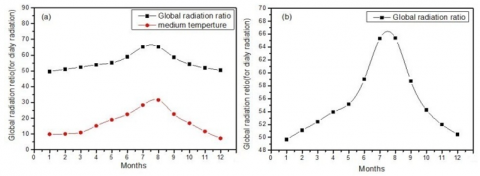Figure 7. (a) the Variations of (H / H0) and T temperature versus months of 2018, (b) The variation of H / H0 changes in terms of months of 2018

## 8.png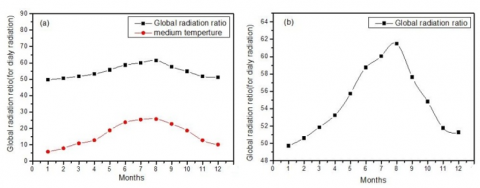Figure 8. (a) The variations of (H / H0) and T temperature versus months of the year 2019, (b) The variation of H / H0 changes in terms of months of 2019

## 9.pngFigure 9. The variations of (H / H0) and T temperature versus months of 2020, (b) The variation of H / H0 changes in terms of months of 2020

As we can see in the Figures (6, 7, 8, 9), the range of H/H0 calculated for each day of the month of the four years (2017-2020) follows that of the temperatures.

5. Results and Analysis

To validate our model, we conducted a study for different sites in Algeria with different climates. Daily data, concerning the values of the average temperature T and the relative humidity RH, were collected for the year 2020, for several cities in Algeria. These data are taken from GSOD and CDER. The geographical characteristics of each city (latitude, longitude and elevation above sea level) are taken from the center of renewable energy development. For our calculations, we chose a typical day for each season, the 20th of February for winter (n=51), the 20th of April for spring (n=110) the 20th of July for summer (n=201) and the 20th of November for the fall (n = 324).

We calculated the extraterrestrial solar irradiance on a horizontal surface $\mathrm{H}_{0}$ (W/m²) for each selected typical day. Then, we used our proposed new model for the calculation of the global solar radiation $\mathrm{H}_{\text {Calculated }}$ for each zone, as shown in Table 6.

Table 6. The data of longitude, the sun declination, height, temperature; the average humidity, the ratio of global radiation to daily radiation form CDER DATABASES compared with our results which are obtained by our proposed model

 State Date Day Number in the year Ø[°] Δ[°] h(m) T[°C] RH $\mathrm{H}_{0}$ (W/m2) $\frac{H_{\text {calculated }}}{H_{0}}$ $\frac{H_{C D E R}}{H_{0}}$ $H_{\text {calculated }}$ (W/m2) $H_{C D E R}$ (W/m2) $\Delta H / H_{\text {Calculated }}$ The average value of $\Delta H / H_{\text {Calculated }}(\%)$ 20/02/2020 51 7.95 -11.22 680 10.8 0.688 181.36 52.18 56.29 9463.36 10209 7.87 Souk Ahras 20/04/2020 110 7.95 11.23 680 13,5 0.66 175.04 64.83 68.45 11348.88 11983 5.58 20/07/2020 201 7.95 20.82 680 27.3 0.472 171.44 68.42 67.64 10869.2 11597 6.69 6.49 20/11/2020 324 7.95 -19.52 680 9,9 0.904 160.64 53.41 50.29 8580 8079 5.83 20/02/2020 51 -0.28 -11.22 263 18.4 0.233 181.9 60.16 56.15 10943.1 10215 6.65 Adrar 20/04/2020 110 -0.28 11.23 263 23.8 0.545 175.95 60.09 64.23 10572.83 11303 6.9 20/07/2020 201 -0.28 20.82 263 39.2 0.125 138.47 78.32 76.65 10845.15 10615 2.12 4.71 20/11/2020 324 -0.28 -1952 263 17.1 0.412 158.65 57.6 55.75 9138.24 8846 3.19 20/02/2020 51 0.12 -11.22 137 16.9 0.646 177.91 55.02 53.4 9788.6 9501 2.93 20/04/2020 110 0.12 11.23 137 21 0.455 186.17 59.36 60.14 11051.05 11197 1.32 Mostaganim 20/07/2020 201 0.12 20.82 137 31.9 0.474 164.87 67.26 65.32 11087.5 10770 2.86 2.49 20/11/2020 324 0.12 -19.52 137 15.3 0.832 174.09 52.32 44.09 7899.1 7674 2.85 20/02/2020 51 -0.6 -11.22 90 13.2 0.761 185.46 52.22 50.97 9684.72 9453 2.39 20/04/2020 110 -0.6 11.23 90 20.9 0.677 185.76 56.83 59.32 10556.74 11020 4.38 Oran/Senia 20/07/2020 201 -0.6 20.82 90 26.5 0.666 163.58 60.72 65.29 9933.67 10681 7.52 5.72 20/11/2020 324 -0.6 -19.52 90 14.6 0.725 175.98 53.16 43.39 8355.09 7636 8.6 20/02/2020 51 8.13 -11.22 813 11.6 0.527 184.89 58.7 56.35 10853.04 10420 4 20/04/2020 110 8.13 11.23 813 18.5 0.456 188.86 58.14 64.3 10980.32 12144 10.59 Tebessa 20/07/2020 201 8.13 20.82 813 26.5 0.393 170.91 63.62 68.63 10873.29 11730 7.87 6.82 20/11/2020 324 8.13 -19.52 813 9.7 0.844 164.82 50.2 52.63 8273.26 8675 4.84 20/02/2020 51 7.82 -11.22 4 11.3 0.764 176.81 51.52 52.35 9109.25 9259 1.64 Annaba 20/04/2020 110 7.82 11.23 4 18.7 0.922 189.2 53 58.21 10027.6 11014 9.83 20/07/2020 201 7.82 20.82 4 24.4 0.739 170.71 58.44 62.21 9976.29 10621 6.46 6.85 20/11/2020 324 7.82 -19.52 4 16.3 0.704 165.32 54.12 43.49 7947.11 7191 9.5 20/02/2020 51 5,52 -11.22 1378 16,9 0.446 180.65 57.17 64.09 10327.76 11579 12.11 20/04/2020 110 5.52 11.23 1378 26.6 0.207 178.69 65.88 69.86 11736.35 12484 6.37 Tamanrasset 20/07/2020 201 5.52 20.82 1378 32.5 0.164 169.56 71.08 68.6 12052.32 11632 3.48 7.19 20/11/2020 324 5.52 -19.52 1378 16.8 0.263 168.66 59.06 63.09 9961.05 10641 6.82 20/02/2020 51 5.42 -11.22 1038 6 0.505 177.6 52.79 60.1 9407.47 10675 13.47 20/04/2020 110 5.42 11.23 1038 17.2 0.422 188.61 56.68 66.08 11690.41 12464 6.61 Setif 20/07/2020 201 5.42 20.82 1038 25.8 0.296 169.46 64.13 71.32 10867.46 12097 11.3 8.93 20/11/2020 324 5.42 -19.52 1038 7,6 0.882 180.44 49.28 47.13 8892.08 8505 4.35 20/02/2020 51 3.82 -11.22 450 13.6 0.442 179.01 55.74 57.05 9978.01 10213 2.35 Gherdaia 20/04/2020 110 3.82 11.23 450 22,8 0.466 187.94 60.26 62.05 11325.26 11662 2.97 20/07/2020 201 3.82 20.82 450 32.7 0.258 167.27 70.27 66.09 11754.06 11055 5.94 5.36 20/11/2020 324 3.82 -19.52 450 15.6 0.486 171.11 56.12 50.38 9602.69 8622 10.21 20/02/2020 51 5.4 -11.22 141 14.2 0.481 180.66 55.58 54.73 10041.08 9888 1.52 20/04/2020 110 5.4 11.23 141 27.8 0.465 178.62 61.72 63.02 11579.9 11257 2.78 Ouergla 20/07/2020 201 5.4 20.82 141 30.1 0.239 169.43 68.16 62.86 11548.34 10651 7.77 6.04 20/11/2020 324 5.4 -19.52 141 16.1 0.541 168.87 56.12 49 9416.19 8276 12.1

We compared our values of $\left(\mathrm{H}_{\text {Calculated }} / \mathrm{H}_{0}\right)$ computed using the proposed model for each typical day of each season for each chosen city, with the values of $\left(\mathrm{H}_{\mathrm{CDER}} / \mathrm{H}_{0}\right)$ taken from the CDER database. We note that the smaller the value of $\Delta \mathrm{H} / \mathrm{H}_{\text {Calculated }}$, the more precise the value of $\mathrm{H}$ calculated according to the proposed model.

If we take the case of Souk Ahras (extreme east of the country), the value of $\Delta H / H_{\text {Calculated }}$ is $6.49 \%$. The others are $4.71 \%$ for Adrar (south west of the Algeria), $2.49 \%$ for Mostaganem and $5.72 \%$ for Oran. For the cities of the Algerian Sahara, the value of $\Delta \mathrm{H} / \mathrm{H}_{\text {Calculated }}$ is between $5.36 \%-7.19 \%$ and it is $8.93 \%$ for Setif city. Given these values, it can be confirmed that the proposed model gives very satisfactory results for the evaluation of global solar radiation across the country of Algeria.

This model shows the global solar radiation reaching the Earth’s surface affected by several factors. The most important of which are:

• The nature of gaseous envelope and the substances suspended in it.
• The angle at which the sun’s rays reach the earth.
• The length of time during which the sun shines and this change according to the seasons.
• The nature of the region's climate.
• The temperature decreases during the short day and rises during the long day. It’s also affected by latitude, which causes a difference in temperature.

From the Table 6 we draw graphs (H/H0 Calculated), (H/H0 Taken for CDER) as the following (Figure 10, Figure 11, Figure 12, Figure 13, Figure 14, Figure 15, Figure 16, Figure 17, Figure 18, Figure 19).

## 10.pngFigure 10. The variation of Hcal / H0 and HCDER / H0 in terms of months in Souk Ahras

## 11.png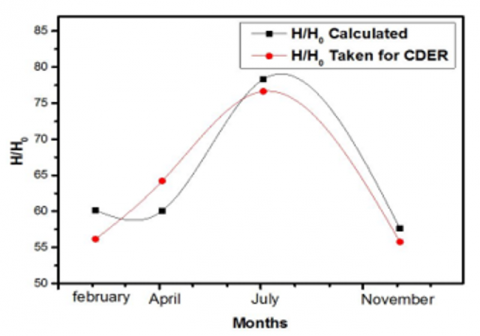Figure 11. The variation of Hcal / H0 and HCDER / H0 in terms of months in Adrar

## 12.pngFigure 12. The variation of Hcal / H0 and HCDER / H0 in terms of months in Mostaganem

## 13.png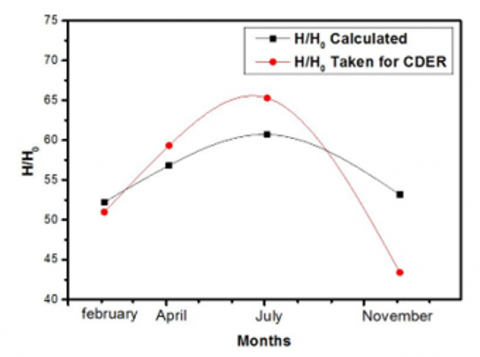Figure 13. The variation of Hcal / H0 and HCDER / H0 in terms of months in Oran/Senia

## 14.pngFigure 14. The variation of Hcal / H0 and HCDER / H0 in terms of months in Tebessa

## 15.png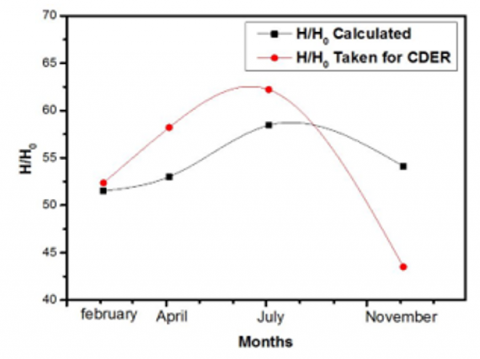Figure 15. The variation of Hcal / H0 and HCDER / H0 in terms of months in Annaba

## 16.png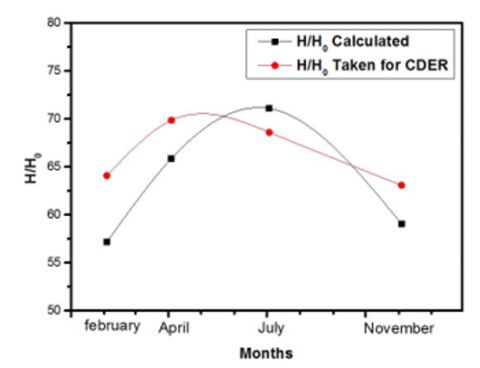Figure 16. The variation of Hcal / H0 and HCDER / H0 in terms of months in Tamanrasset

## 17.pngFigure 17. The variation of Hcal / H0 and HCDER / H0 in terms of months in Setif

## 18.png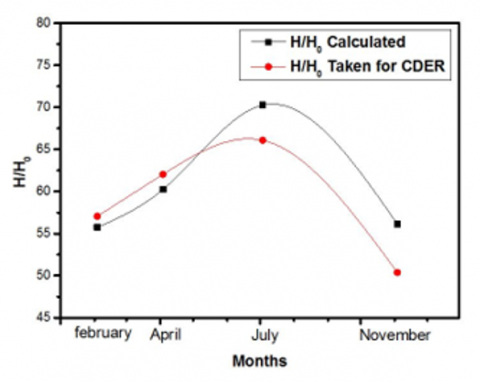Figure 18. The variation of Hcal / H0 and HCDER / H0 in terms of months in Ghardaia

## 19.png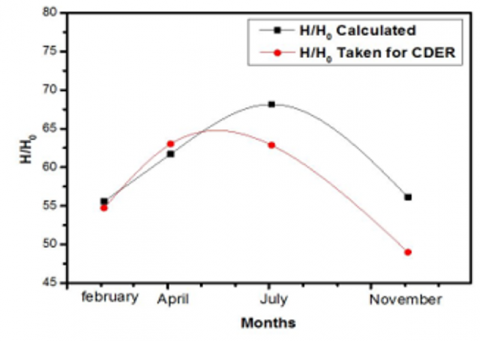Figure 19. The variation of Hcal/ H0 and HCDER / H0 in terms of months in Ouergla

6. Conclusion

The future of the use of solar energy, which is a renewable and sustainable energy, requires accurate information about solar radiation and its components in any place on earth. That is why, the modeling of solar radiation for its evaluation or prediction is of great importance, especially in areas where there are no measured values. The main goal of this work is to propose a new model to estimate the global solar radiation for the region of Souk Ahras in Algeria especially that direct measurement data are difficult to obtain or even impossible to find because of the lack of means and stations for climatic and meteorological measurements in that area.

In this article, we presented a literature review on various existing models based on the regression equation and the duration of sunshine or on the temperature or even hybrid models based on several parameters for the calculation of global solar radiation for several regions in the world. We noticed that almost every model is proposed for a given area because of the diversity of climate, meteorological and geographical parameters.

The proposed model is made on data collection over four years (2017-2020). These data relate to the duration of sunshine, the daily temperature per hour of the air, the daily relative humidity and the geographical coordinates taken from the database (GOSD) and from (CDER). Based on the regression equation, we arrived at estimating the regression coefficients a, b and c specific to the Souk Ahras region. We made a comparison of the calculation result of the global solar radiation of several other sites in Algeria made from our model $\mathrm{H}_{\text {calculated }}$) and those experimental proposed by the center for the development of renewable energies ( $\mathrm{H}_{\text {CDER }}$ ). We found that there is a good agreement between the results and that the proposed model has a very acceptable precision for the majority of the cities considered.

The model proposed in this study can be used anywhere in the world where climatic conditions are the same.

Nomenclature
 H the monthly average daily global solar irradiation on horizontal surface )W/m2) H0 the extra-terrestrial solar radiance on a horizontal surface )W/m2) Hc the monthly average clear sky daily global radiation )W/m2) Hd the monthly mean daily diffuse solar irradiance on a horizontal surface )W/m2) Isc the solar constant (W/m2) δ the solar declination (°) N the number of days of the year starting from first January S the monthly average daily bright sunshine duration (hours) S0 The day length (hours). ws the sunset hour angle (°) $\varnothing$ the latitude of location (°) RH the mean relative humidity (%) T Average temperature(°C) ∆T the temperature term difference (°C).
References

 Ministry of Energy and Mines. http://www.energy.gov.dz/?rubrique=energies-nouvelles-renouvelables-et-maitrise-de-lrenergie.

 Renewables Now. http://renewablesnow.com/news/algeria-selects-11-sites-for-1-gw-solar-project-762545.

 https://fr.wikipedia.org/wiki/Souk_Ahras#cite_note-4,

 Li, D.H., Lou, S.W., Lam, J.C. (2015). An analysis of global, direct and diffuse solar radiation. Energy Proceedia, 75: 388-393. https://doi.org/10.1016/j.egypro.2015.07.399

 Karfoul, R. (2019). The calculation and the analysis of the quantity of the total solar radiation incident on tilted surface on a high region above sea level. Tishreen University Journal-Basic Sciences Series, 41(4).

 Muzathik, A.M., Nik, W.B.W., Ibrahim, M.Z., Samo, K.B., Sopian, K., Alghoul, M.A. (2011). Daily global solar radiation estimate based on sunshine hours. International Journal of Mechanical and Materials Engineering, 6(1): 75-80.

 Ayodele, T.R., Ogunjuyigbe, A.S.O. (2015). Prediction of monthly average global solar radiation based on statistical distribution of clearness index. Energy, 90: 1733-1742. https://doi.org/10.1016/j.energy.2015.06.137

 Glover, J., McCulloch, J.S.G. (1958). The empirical relation between solar radiation and hours of sunshine. Quarterly Journal of the Royal Meteorological Society, 84(360): 172-175. https://doi.org/10.1002/qj.49708436011

 Page, J.K. (1961). The estimation of monthly mean values of daily total short wave radiation on-vertical and inclined surfaces from sun shine records for latitudes 400 N–400 S. In Proceedings of the United Nations Conference on New Sources of Energy, 98(4): 378-390. https://digitallibrary.un.org/record/3827996.

 Swartman, R.K., Ogunlade, O. (1967). Solar radiation estimates from common parameters. Solar Energy, 11(3-4): 170-172. https://doi.org/10.1016/0038-092X(67)90026-6

 Iqbal, M. (1979). Correlation of average diffuse and beam radiation with hours of bright sunshine. Solar Energy, 23(2): 169-173. https://doi.org/10.1016/0038-092X(79)90118-X

 Khogali, A., Ramadan, M.R.I., Ali, Z.E.H., Fattah, Y.A. (1983). Global and diffuse solar irradiance in Yemen (YAR). Solar Energy, 31(1): 55-62. https://doi.org/10.1016/0038-092X(83)90033-6

 Benson, R.B., Paris, M.V., Sherry, J.E., Justus, C.G. (1984). Estimation of daily and monthly direct, diffuse and global solar radiation from sunshine duration measurements. Solar Energy, 32(4): 523-535. https://doi.org/10.1016/0038-092X(84)90267-6

 Ögelman, H., Ecevit, A., Tasdemiroǧlu, E. (1984). A new method for estimating solar radiation from bright sunshine data. Solar Energy, 33(6): 619-625. https://doi.org/10.1016/0038-092X(84)90018-5

 Bahel, V., Srinivasan, R., Bakhsh, H. (1986). Solar radiation for Dhahran, Saudi Arabia. Energy, 11(10): 985-989. https://doi.org/10.1016/0360-5442(86)90029-0

 Bahel, V., Bakhsh, H., Srinivasan, R. (1987). A correlation for estimation of global solar radiation. Energy, 12(2): 131-135. https://doi.org/10.1016/0360-5442(87)90117-4

 Gopinathan, K.K. (1988). A general formula for computing the coefficients of the correlation connecting global solar radiation to sunshine duration. Solar Energy, 41(6): 499-502. https://doi.org/10.1016/0038-092X(88)90052-7

 Newland, F.J. (1989). A study of solar radiation models for the coastal region of South China. Solar Energy, 43(4): 227-235. https://doi.org/10.1016/0038-092X(89)90022-4

 Alsaad, M.A. (1990). Characteristic distribution of global solar radiation for Amman, Jordan. Solar & Wind Technology, 7(2-3): 261-266. https://doi.org/10.1016/0741-983X(90)90095-J

 Akinoğlu, B.G., Ecevit, A. (1990). A further comparison and discussion of sunshine-based models to estimate global solar radiation. Energy, 15(10): 865-872. https://doi.org/10.1016/0360-5442(90)90068-D

 Samuel, T.D.M.A. (1991). Estimation of global radiation for Sri Lanka. Solar Energy, 47(5): 333-337. https://doi.org/10.1016/0038-092X(91)90026-S

 Lewis, G. (1992). An empirical relation for estimating global irradiation for Tennessee, USA. Energy Conversion and Management, 33(12): 1097-1099. https://doi.org/10.1016/0196-8904(92)90007-J

 Aksoy, B. (1997). Estimated monthly average global radiation for Turkey and its comparison with observations. Renewable Energy, 10(4): 625-633. https://doi.org/10.1016/S0960-1481(96)00035-3

 Ampratwum, D.B., Dorvlo, A.S. (1999). Estimation of solar radiation from the number of sunshine hours. Applied Energy, 63(3): 161-167. https://doi.org/10.1016/S0306-2619(99)00025-2

 Chegaar, M., Chibani, A. (2001). Global solar radiation estimation in Algeria. Energy Conversion and Management, 42(8): 967-973. https://doi.org/10.1016/S0196-8904(00)00105-9

 Akpabio, L.E., Etuk, S.E. (2003). Relationship between global solar radiation and sunshine duration for Onne, Nigeria. Turkish Journal of Physics, 27(2): 161-167.

 Ahmed, F., Ulfat, I. (2004). Empirical models for the correlation of monthly average daily global solar radiation with hours of sunshine on a horizontal surface at Karachi, Pakistan. Turkish Journal of Physics, 28(5): 301-307.

 Chen, R., Ersi, K., Yang, J., Lu, S., Zhao, W. (2004). Validation of five global radiation models with measured daily data in China. Energy Conversion and Management, 45(11-12): 1759-1769. https://doi.org/10.1016/j.enconman.2003.09.019

 Bakirci, K. (2007). Estimation of global solar radiation on horizontal surface. Journal of Thermal Science and Technology, 27(1): 7-11.

 Bakirci, K. (2009). Estimation of solar radiation by using ASHRAE clear-sky model in Erzurum, Turkey. Energy Sources, Part A, 31(3): 208-216. https://doi.org/10.1080/15567030701522534

 Olayinka, S. (2011). Estimation of global and diffuse solar radiations for selected cities in Nigeria. International Journal of Energy and Environmental Engineering, 2(3): 13-33.

 Adaramola, M.S. (2012). Estimating global solar radiation using common meteorological data in Akure, Nigeria. Renewable Energy, 47: 38-44. https://doi.org/10.1016/j.renene.2012.04.005

 Adhikari, K.R., Bhattarai, B.K., Gurung, S. (2013). Estimation of global solar radiation for four selected sites in Nepal using sunshine hours, temperature and relative humidity. Journal of Power and Energy Engineering, 1(03): 1. http://doi.org/10.4236/jpee.2013.13003

 Li, M.F., Tang, X.P., Wu, W., Liu, H.B. (2013). General models for estimating daily global solar radiation for different solar radiation zones in mainland China. Energy Conversion and Management, 70: 139-148. https://doi.org/10.1016/j.enconman.2013.03.004

 Hassan, G.E., Youssef, M.E., Mohamed, Z.E., Ali, M.A., Hanafy, A.A. (2016). New temperature-based models for predicting global solar radiation. Applied Energy, 179: 437-450. https://doi.org/10.1016/j.apenergy.2016.07.006

 Jahani, B., Dinpashoh, Y., Nafchi, A.R. (2017). Evaluation and development of empirical models for estimating daily solar radiation. Renewable and Sustainable Energy Reviews, 73: 878-891. https://doi.org/10.1016/j.rser.2017.01.124

 Fan, J., Chen, B., Wu, L., Zhang, F., Lu, X., Xiang, Y. (2018). Evaluation and development of temperature-based empirical models for estimating daily global solar radiation in humid regions. Energy, 144: 903-914. https://doi.org/10.1016/j.energy.2017.12.091

 Ekici, C., Teke, I. (2018). Developing a new solar radiation estimation model based on Buckingham theorem. Results in Physics, 9: 263-269. https://doi.org/10.1016/j.rinp.2018.02.064

 Yıldırım, H.B., Teke, A., Antonanzas-Torres, F. (2018). Evaluation of classical parametric models for estimating solar radiation in the Eastern Mediterranean region of Turkey. Renewable and Sustainable Energy Reviews, 82: 2053-2065. https://doi.org/10.1016/j.rser.2017.08.033

 Duffie, J.A., Beckman, W.A. (1991). Solar Engineering of Thermal Processes, New York. Chichester, Brisbane, Toronto, Singapore. https://doi.org/10.1119/1.14178

 Renewable Energy Development Center. http://www.cder.dz/.

 Global Surface Summary of Day. http://www.ogimet.com/gsodc.phtml.en.